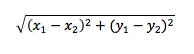## Problems from previous (non-Python) introductory courses

In addition, to writing and submitting several programs from the following list. There have been typically short answer and multiple choice questions on problem solving, programming, and code analysis. Problems that involve slicing and ranging seem essentially appropriate (but not guaranteed) for a class using Python. Please be aware that class personnel never provide answers to past test programming problems.

• Produce a program that determines the walking distance between to Manhattan locations gotten from the program user.
• Produce a program that determines whether the relationship between two integer inputs has the first input less than the second input, the first input equal to the second input, or the first input greater than the second input.
• Produce a program that gets a single decimal input. The input is the distance in kilometers of a satellite from the surface of the earth. The program determines the velocity needed for the satellite to stay in orbit.
• Produce a program that gets two integer inputs: the number of cups drank on a normal weekday; and second, the number of cups of coffee drank on a normal weekend day. The program determines the number of cups drank per 52-week year.
• Produce a program that gets the name of a non-empty web file whose from input and determines when ignoring case whether all words are the same.
• Produce a program that examines a non-empty web file, where each line in the file contains three values: the name of a country, the average life expectancy of a person from that country, and the expected yearly ecological footprint of a person from that country. The per capita lifetime footprint for a country is the product of those two measures. For example, the per capita lifeprint of a Costa Rican is 198.2 (79.3 × 2.5); and the per capita lifetime footprint of a Vietnamese is 105.2 (75.2 × 1.4). Determine the countries with the lowest and highest per capita lifetime footprints and their values.
• Produce a program that prints your favorite color.
• Produce a program that determines the perimiter of a circle whose radius is gotten from the program user.
• Produce a program that gets a Boolean value from its user. If the user enters true, the program prints false; else if instead the user enters false, the program prints true; else the program prints an error message.
• Produce a program that determines whether a string is a palindrome; that is, it reads the same in forward and backward directions.
• Produce a program that determines whether a word gotten from input is voweless.
• Produce a program that determines the energy equivalent of a mass value gotten from input.
• Produce a program that prints a line of input stripped of all vowels.
• Produce a program that takes two integer inputs b and n. The program displays on a single line the values of the multiples 0 × b, 1 × b, 2 × b, …, n × b. The only thing on the line should be the numbers and spaces.
• Produce a program that determines the longest word in a web-based data set.
• Produce a program that gets four decimal inputs x1, y1, x2, and y2 in that order. The program computes the decimal distance between locations (x1, y1) and (x2, y2). The distance between the locations is given by formula• Produce a program that gets a single integer input. The program determine whether the input is a legal minute value for a clock (i.e., at least 0 and at most 59). For legal minute values, the program prints out the next clock minute. For illegal minute values, the program prints out an error message. There should be no other output.
• Produce a program that takes four integer inputs a, b, c, and d. Value a is used to intialize a random number generator. Value b is a count. The program generates b random numbers from the range ( c, d ).
• Produce a program that gets two lines of input, the program determine which line is longer.
• Produce a program that gets two lines of integer input. The program reports whether the two lines represent the same values in possbily different order. To be duplicates, the sorted versions of the arrays must be equal to each other.
• Produce a program that take the name of a web file as input. The program displays the number of words in the web file and their average word length
• Produce a program that takes two lines of input. The program reports when stripped of whitespace whether the lines represent same character string.